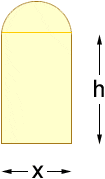# I suck at word problems .

I suck at word problems :(.

I was wondering if someone could look over how I’m setting up this word problem. I’m not sure if I’m doing it correctly.

## Homework Statement

A Norman window has the shape of a rectangle surmounted by a semicircle. If the perimeter of the window is 30ft, express the area A of the window as a function of the width of the window.

P = 30

## Homework Equations

To find the area A of a rectangle multiply it’s width W by its length L.

1) A = WL

The perimeter of a rectangle is equal to sum of its widths and its lengths.
2) P = 2L+2W

## The Attempt at a Solution

30 = 2L + 2W
2L = 30 – 2W
L = (30 – 2W) /2

Let the width W of the rectangle equal x.
3) A(x) = x * L

Then,
A(x) = x((30 – 2x)/2)

Hmm...it seems that you have not taken the semi-circle into consideration.

Perimeter, in simple terms, is the length of the border of a geometrical figure. Here, you have a semi-circle sitting on top of a rectangle. What is its perimeter?

cristo
Staff Emeritus

## Homework Statement

A Norman window has the shape of a rectangle surmounted by a semicircle If the perimeter of the window is 30ft, express the area A of the window as a function of the width of the window.

Re-read the bit in red. The window has the shape of a rectangle with a semicircle attached to the top. Can you write an equation for the perimeter of such a shape?

wow, I totally missed that part and I read the problem like 5 times... add for the loss :(.

wow, I totally missed that part and I read the problem like 5 times... add for the loss :(.

First you need to take into account that the semicircle is included as part of the perimeter, you need to do that first to find the area of the rectangle portion.

Ok, the radius of the semi-circle is equal to the width of the rectangle / 2.

Then to find the area:
$$A(x) = x((30-2x)/2) + (\PI(x/2)^2)/2$$

Last edited:
What's that w?

sorry, that was supposed to be an x. The width of the rectangle = x

HallsofIvy
Homework Helper
You will also have to realize that the edge of the rectangle that the semicircle is sitting on is NOT part of the perimeter!

You will also have to realize that the edge of the rectangle that the semicircle is sitting on is NOT part of the perimeter!

I'm not sure I understand what you mean...

danago
Gold MemberSee where the semi-circle and rectangle join? That line they have in common is not part of the perimeter. Only the outside of the overall shape forms the perimeter.

... ok now I'm lost :(

Is there something else I need to add to the equation in order for it to be correct?

$$A(x) = x((30-2x)/2) + (\PI(x/2)^2)/2$$

I have no idea how to calculate the for the line in between.

cristo
Staff Emeritus
Your calculation for the area is incorrect (n.b. the command for pi in LaTex is "\pi")

I suspect you have calculated the perimeter incorrectly, and thus have the incorrect expression for h in terms of x. Try calculating the perimeter again, noting that it consists of two sides of the rectangle, the bottom of the rectangle and the semicircular arc on top.

arildno
Homework Helper
Gold Member
Dearly Missed
I was wondering if someone could look over how I’m setting up this word problem. I’m not sure if I’m doing it correctly.

## Homework Statement

A Norman window has the shape of a rectangle surmounted by a semicircle. If the perimeter of the window is 30ft, express the area A of the window as a function of the width of the window.

In order to solve word problems, take care to define all relevant quantities first, unkown and knowns.
Then proceed to set up relations, that is equations, among them, if you haven't gotten them already!

I'll start this for you:
1. W-width of rectangular section of window (unknown quantity)
2. H- height of rectangular section of window (unknown quantity)
3. R- radius of semi-circular section of window (unkown quantity)
4. P-perimeter of window (known quantity)
5. A-area of window (unkown quantity)

Aim:
To express A as a function of W, i.e, find A(W)

Auxiliary relations:
1. Radius of circle equals half the width, i.e, $$R=W/2 (1)$$
2. Perimeter of window consists of two parts: the perimeter of the rectangular section minus 1 width, PLUS the length of the circular arc (which is half the circumference of a circle).
Thus, knowing that this totals 30, we get our equation for the perimeter:
$$W+2H+\pi{R}=30 (2)$$
3. The area A consists of two parts, the area of the rectangular piece, and the area of of the semi-circular piece.
Thus, we get the equation for A:
$$A=WH+\frac{\pi{R}^{2}}{2}(3)$$

Now, use equations (1) and (2) to express R and H in terms of W, and insert your results into (3) to gain the desired expression for A(W).

ah! thanks :)

Last edited: